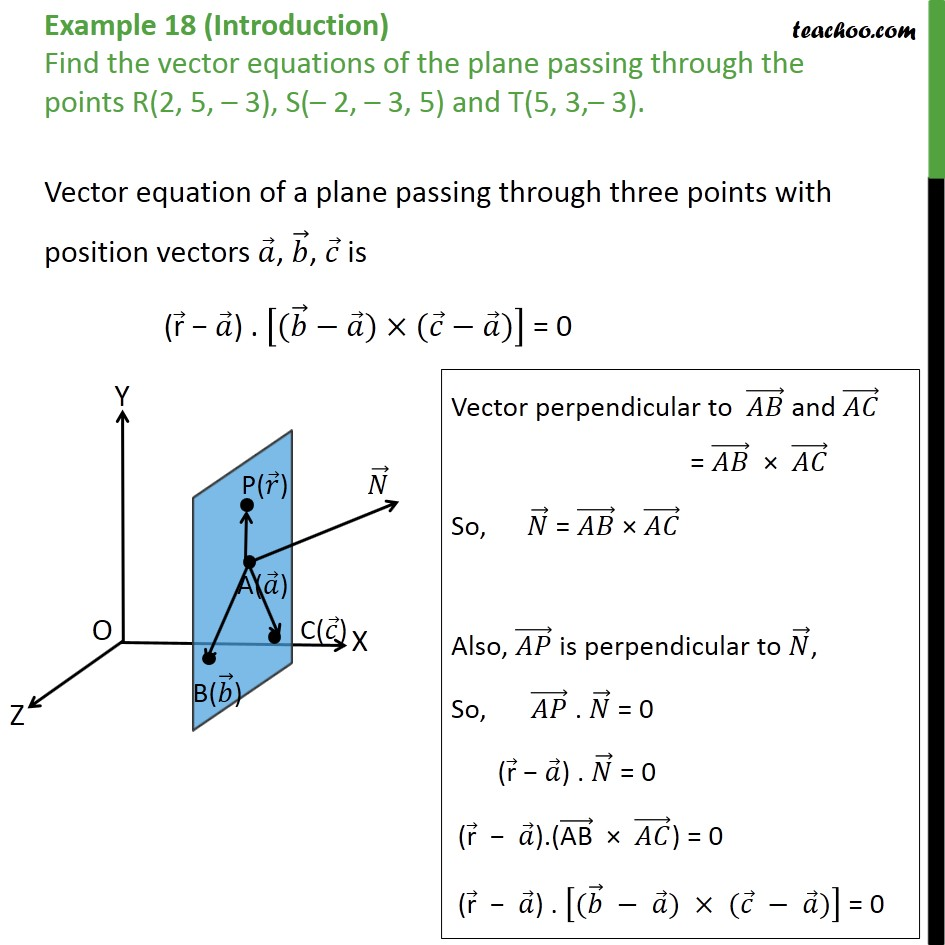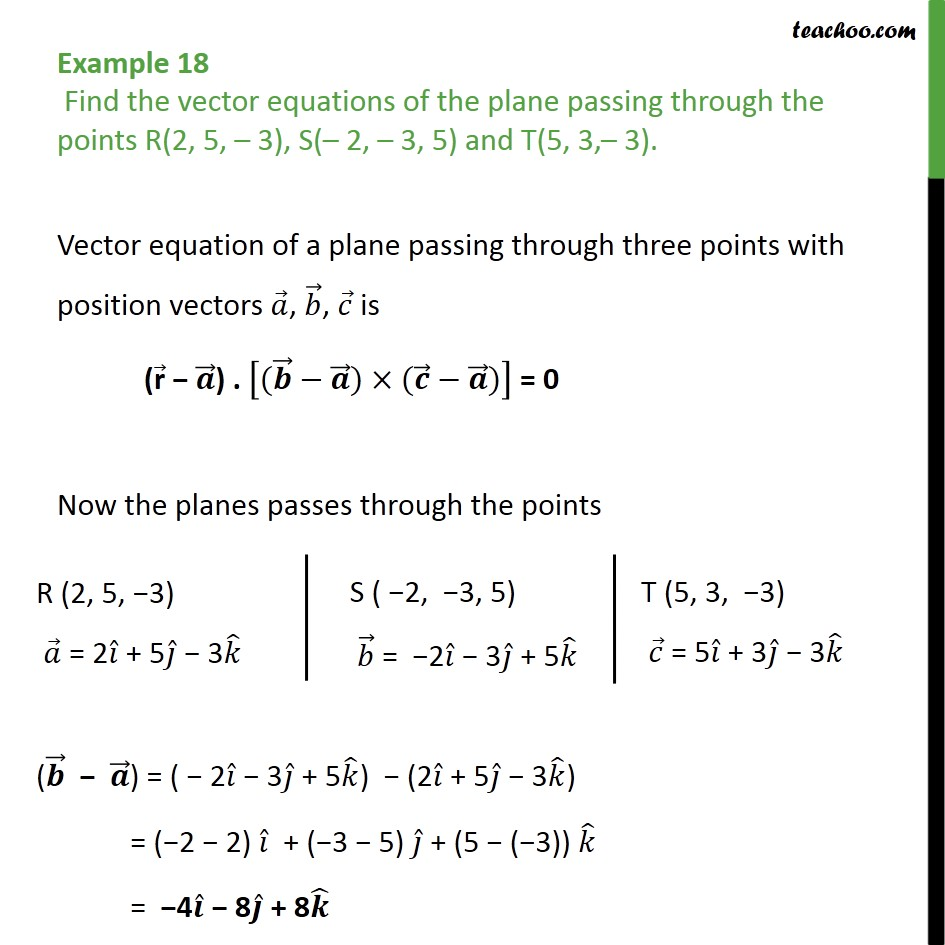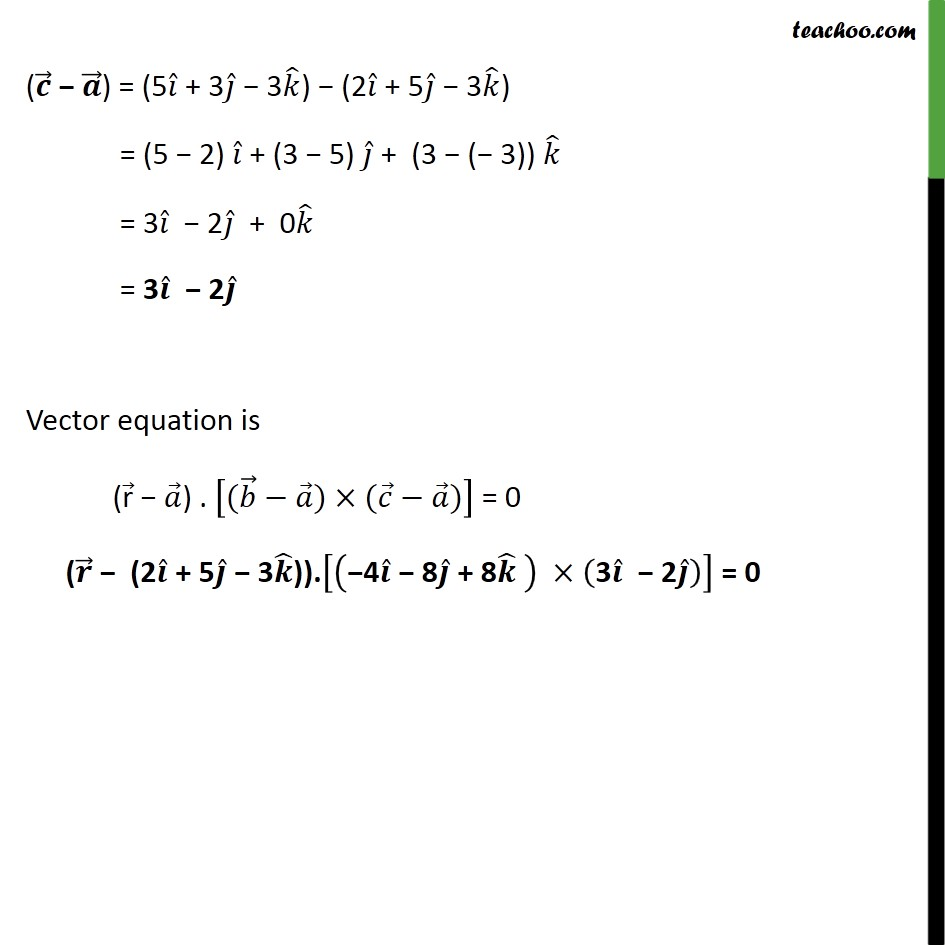1. Chapter 11 Class 12 Three Dimensional Geometry
2. Concept wise
3. Equation of plane - Passing Through 3 Non Collinear Points

Transcript

Example 18 (Introduction) Find the vector equations of the plane passing through the points R(2, 5, 3), S( 2, 3, 5) and T(5, 3, 3). Vector equation of a plane passing through three points with position vectors , , is ( r ) . ( ) ( ) = 0 Example 18 Find the vector equations of the plane passing through the points R(2, 5, 3), S( 2, 3, 5) and T(5, 3, 3). Vector equation of a plane passing through three points with position vectors , , is ( r ) . ( ) ( ) = 0 Now the planes passes through the points ( ) = (5 + 3 3 ) (2 + 5 3 ) = (5 2) + (3 5) + (3 ( 3)) = 3 2 + 0 = 3 2 Vector equation is ( r ) . ( ) ( ) = 0 ( (2 + 5 3 )). 4 8 + 8 3 2 = 0

Equation of plane - Passing Through 3 Non Collinear Points

About the AuthorDavneet Singh
Davneet Singh is a graduate from Indian Institute of Technology, Kanpur. He has been teaching from the past 9 years. He provides courses for Maths and Science at Teachoo.